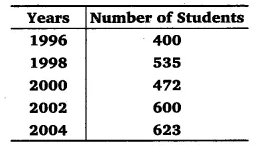# Total number of students of a school in different years is shown in the following table:

Total number of students of a school in different years is shown in the following table:A. Prepare a pictograph of students using one symbol A to represent 100 students and answer the following questions:
(a) How many symbols represent total number of students in the year 2002?
(b) How many symbols represent total number of students for the year 1998?
B. Prepare another pictograph of students using any other symbol each representing 50 students. Which pictograph do you find more informative?